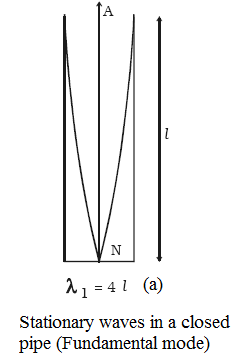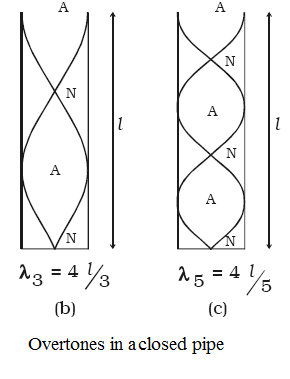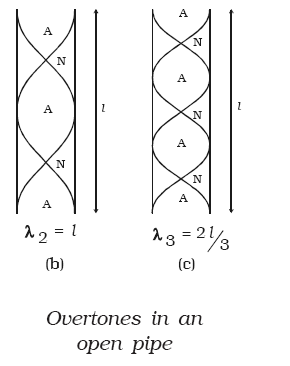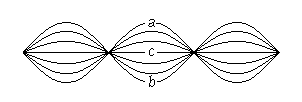Click to Chat

1800-1023-196

+91-120-4616500

CART 0

• 0

MY CART (5)

Use Coupon: CART20 and get 20% off on all online Study Material

ITEM
DETAILS
MRP
DISCOUNT
FINAL PRICE
Total Price: Rs.

There are no items in this cart.
Continue Shopping• Complete Physics Course - Class 11
• OFFERED PRICE: Rs. 2,968
• View Details

```Vibrations of Air Column in PipesMusical wind instruments like flute, clarinet etc. are based on the principle of vibrations of air columns. Due to the superposition of the incident wave and the reflected wave, longitudinal stationary waves are formed in the pipe.

Organ pipes

Organ pipes are musical instruments which are used to produce musical sound by blowing air into the pipe. Organ pipes are two types (a) closed organ pipes, closed at one end (b) open organ pipe, open at both ends.

(a) Closed organ pipe

If the air is blown lightly at the open end of the closed organ pipe, then the air column vibrates (as shown in figure) in the fundamental mode. There is a node at the closed end and an antinode at the open end. If l is the length of the tube,

l = λ1/4 or λ1 = 4l               …... (1)

If n1 is the fundamental frequency of the vibrations and v is the velocity of sound in air, then

n1 = v/λ1 = v/4l                  …... (2)

If air is blown strongly at the open end, frequencies higher than fundamental frequency can be produced. They are called overtones. Fig.b & Fig.c shows the mode of vibration with two or more nodes and antinodes.l = 3λ3/4     or λ3 = 4l/3              …... (3)

Thus, n3 = v/λ3 = 3v/4l = 3n1      …... (4)

This is the first overtone or third harmonic.

Similarly, n5 = 5v/4l = 5n1          …... (5)

This is called as second overtone or fifth harmonic.

Therefore the frequency of pth overtone is (2p + 1) n1 where n1 is the fundamental frequency. In a closed pipe only odd harmonics are produced. The frequencies of harmonics are in the ratio of 1 : 3 : 5.....

(b) Open organ pipe

When air is blown into the open organ pipe, the air column vibrates in the fundamental mode as shown in figure. Antinodes are formed at the ends and a node is formed in the middle of the pipe. If l is the length of the pipe, thenl = λ1/2    Or   λ1 = 2l                 …... (1)

v = n1λ1 = n12l

The fundamental frequency,

n1 = v/2l                      …... (2)

In the next mode of vibration additional nodes and antinodes are formed as shown in Fig.b and Fig.c.

l = λ2 or v = n2λ2 = n2 (l)

So,  n2 = v/l = 2n1         …... (3)

This is the first overtone or second harmonic.

Similarly,n3 = v/λ3 = 3v/2l = 3n1     …... (4)

This is the second overtone or third harmonic

Therefore the frequency of Pth overtone is (P + 1) n1 where n1 is the fundamental frequency.

The frequencies of harmonics are in the ratio of 1 : 2 : 3 ....

Resonance air column apparatus

The resonance air column apparatus consists of a glass tube G about one metre in length (as shown in figure) whose lower end is connected to a reservoir R by a rubber tube.

The glass tube is mounted on a vertical stand with a scale attached to it. The glass tube is partly filled with water. The level of water in the tube can be adjusted by raising or lowering the reservoir.

A vibrating tuning fork of frequency n is held near the open end of the tube. The length of the air column is adjusted by changing the water level. The air column of the tube acts like a closed organ pipe. When this air column resonates with the frequency of the fork the intensity of sound is maximum.

Here longitudinal stationary wave is formed with node at the water surface and an antinode near the open end. If l1 is the length of the resonating air columnλ/4 = l1 + e                …... (1)

where e is the end correction.

The length of air column is increased until it resonates again with the tuning fork. If l2 is the length of the air column.

3λ/4 = l2 + e              …... (2)

From equations (1) and (2)

λ/2 = (l2 – l1)             …... (3)

The velocity of sound in air at room temperature

v = nλ = 2n (l2 – l1)          …... (4)

Refer this video to know more about vibrations of air column in pipes

End correction

The antinode is not exactly formed at the open end, but at a small distance above the open end. This is called the end correction.

As l1 + e = λ/4     and   l2 + e =  3λ/4

e = (l2 – 3l1)/2

It is found that e = 0.61r, where r is the radius of the glass tube.The resonant frequencies of air columns depend upon the speed of sound in air as well as the length and geometry of the air column.

Longitudinal pressure waves reflect from either closed or open ends to set up standing wave patterns.

Important in the visualization of these standing waves is the location of the nodes and antinodes of pressure and displacement for the air in the columns

A closed ended instrument has one end closed off, and the other end open.

The frequencies of sounds made by these two types of instruments are different because of the different ways that air will move at a closed or open end of the pipe.

Different amounts of a wavelength in a pipe will result in a different frequency being heard.

Because these are the frequencies of the waves that will naturally resonate in the pipes, we call them the resonant frequencies.

The air at the closed end of the pipe must be a node (not moving), since the air is not free to move there and must be able to be reflected back.

There must also be an antinode where the opening is, since that is where there is maximum movement of the air.

The fundamental (first harmonic) for an open end pipe needs to be an antinode at both ends, since the air can move at both ends.

The lowest frequency is called the fundamental frequency or the 1st harmonic. The higher frequencies are called overtones. Integer multiples of the 1st harmonic are labeled as the 2nd, 3rd, etc., harmonics.

Two open organ pipes of fundamental frequencies n1 and n2 are joined in series. The fundamental frequency of the new pipe so obtained will be,

(a) n1 + n2            (b) n1n2/n1 + n2

(c) n1 + n2/2            (d) √(n12 + n22)

Solution:

We know that, n1 = v/2l1

So, l1 = v/2n1

n2 = v/2l2

So, l2 = v/2n2

Now, n = v/2(l1 + l2)

Substituting the values we get,

n = n1n2/n1 + n2

From the above observation we conclude that, option (b) is correct.Question 1A string is fixed at both ends and plucked so it vibrates in a standing wave mode as shown below. Let upward motion correspond to positive velocities. When the string is in position b, the instantaneous velocity of points along the string

(a) is zero everywhere.

(b) is positive everywhere.

(c) is negative everywhere.

(d) depends on the location

Question 2A string is fixed at both ends and plucked so it vibrates in a standing wave mode as shown below. Let upward motion correspond to positive velocities. When the string is in position c, the instantaneous velocity of points along the string

(a) is zero everywhere.

(b) is positive everywhere.

(c) Is negative everywhere.

(d) Depends on the location.

Question 3

A string is stretched between two fixed points. If the tension in the string is increased, the normal mode frequencies

(a) increase.

(b) decrease.

(c) stay the same.

(d) First increase and then decrease

Question 4

An object is vibrating at its natural frequency. Repeated and periodic vibrations of the same natural frequency impinge upon the vibrating object and the amplitude of its vibrations are observed to increase. This phenomenon is known as ____.

(a) beats            (b) resonance

(c) interference    (d) overtone

Question 5

Standing waves are produced in a wire by vibrating one end at a frequency of 100. Hz. The distance between the 2nd and the 5th nodes is 60.0 cm. The wavelength of the original traveling wave is ____ cm.

(a) 50.0              (b) 40.0

(c) 30.0              (d) 20.0Q.1
Q.2
Q.3
Q.4
Q.5

a

d

a

b

b

Related Resources:-

You might like to Doppler effect.

For getting an idea of the type of questions asked, refer the  Previous Year Question Papers.

To read more, Buy study material of Waves comprising study notes, revision notes, video lectures, previous year solved questions etc. Also browse for more study materials on Physics here.
```### Course Features

• 101 Video Lectures
• Revision Notes
• Previous Year Papers
• Mind Map
• Study Planner
• NCERT Solutions
• Discussion Forum
• Test paper with Video Solution